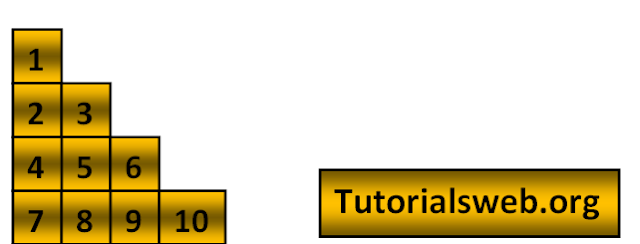## Java Number pattern 19 program using For loop

Write "Java"  program to print below Number Pattern 19:To understand this example, you should have knowledge of the following topics:

·   Java programming operators.

Java Number pattern 19 program using For loop:
`class numberPattern19`
`{`
`public static void main(String[] ags)`
`{`
```   int i,j,k;
k=1;
for(i=1;i<5;i++)
{
for(j=1;j<=i;j++)
{
System.out.print(k++);
}
System.out.println();```
`   }`
` }`
`}`
Output:
`1`
`23`
`456`
`78910`
Explanation:

• First three integers "i", "j" and "k" are declared of type int.
• Then in the First for loop “i” value is initialized with a value 1.
• Now “i” value is checked with the condition i < 5 that is (1 < 5). So the condition is True.
• Now the loop enters into second for loop and checks the condition j <= i. Where "j = 1". and the condition is True.
• Now prints the k value by condition "k++". Print up to second for loop condition becomes False.
• when the value of "i"  becomes "6" the program terminates.